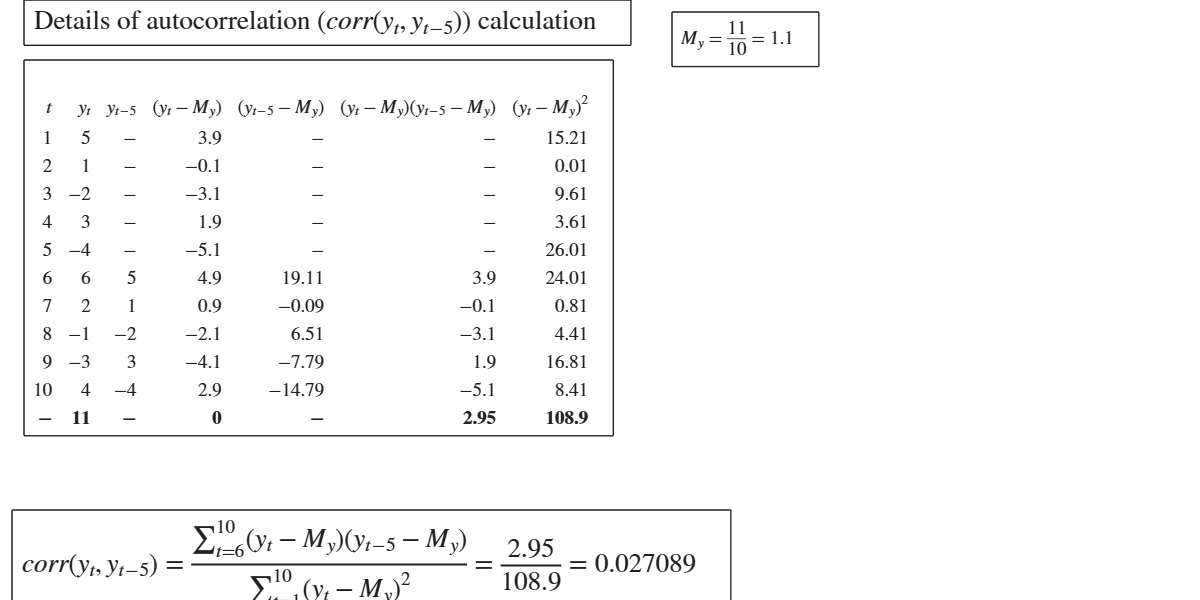# GUIautocorr

GUIautocorr shows the necessary calculations to obtain the autocorrelation in a GUI.

## Syntax

• out=GUIautocorr(y)example
• out=GUIautocorr(y,Name,Value)example

## Description

 out =GUIautocorr(y) Details of calculation of autocorrelation of lag 1.

 out =GUIautocorr(y, Name, Value) Details of calculation of autocorrelation of lag 3.

## Examples

expand all

### Details of calculation of autocorrelation of lag 1.

Imagine we have the following returns for Microsoft prices: Let us suppose we want to compute the autocovariance at lag 1

y=[ 5 1 -2 3 -4 6 2 -1 -3 4];
% If lag is not specified by the default autocorrelation of lag 1 is
% computed
out= GUIautocorr(y);

### Details of calculation of autocorrelation of lag 3.

Imagine we have the following returns for Microsoft prices: Let us suppose we want to compute the autocovariance at lag 1

y=[ 5 1 -2 3 -4 6 2 -1 -3 4];
out= GUIautocorr(y,'lag',3);

## Related Examples

expand all

###Example of use of GUIautocorr when input is a timetable Assume y(1) is first day of march 2023 and the series is daily.

y=[ 5 1 -2 3 -4 6 2 -1 -3 4];
DateStrings = '2023-03-01';
t = datetime(DateStrings,'InputFormat','yyyy-MM-dd');
% Create timetable yt
yt=array2timetable(y','StartTime',t ,'TimeStep',days);
% Compute autocorrelation of lag 5
out= GUIautocorr(yt,'lag',5);## Input Arguments

### y — vector of numeric data or table or timetable. Numeric vector (row or column) or table or timetable.

Vector containing strictly numerical data. If x is table or a timetable the first column of the table (timetable) is used to compute the autocorrelation.

Data Types: vector of doubles or table or timetable

### Name-Value Pair Arguments

Specify optional comma-separated pairs of Name,Value arguments. Name is the argument name and Value is the corresponding value. Name must appear inside single quotes (' '). You can specify several name and value pair arguments in any order as  Name1,Value1,...,NameN,ValueN.

Example:  'lag',2 

### lag —lag.positive scalar.

Vector of the same length of x containing the weights Positive scalar which contains the required lag to compute the autocorrelation. The default is to use lag 1.

Example:  'lag',2 

Data Types: positive scalar

## Output Arguments

### out — description Structure

detailed output to compute the index. struct.

Structure containing the following fields.

Value Description
data

table with T+1 rows (where T is the length of y) containing what is shown in the GUI.

Last row contains the totals.

corr

scalar containing the autocorrelation coefficient for the chosen lag.